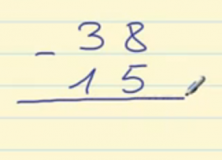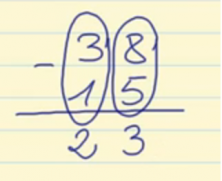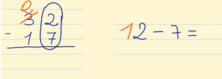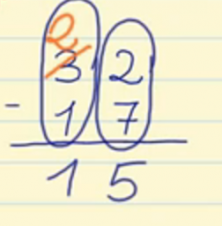Smartick is an advanced online program that teaches kids math and coding in only 15 min. a day
Try it for free!

Jul04

# How to Subtract with and without Borrowing

In this post we will learn to subtract with and without borrowing.

### Subtract Without Borrowing:

The steps we need to follow are:

• Place the number being subtracted under the number being subtracted from so that place values in the same column match.
• Subtract each column separately starting with the ones
• Write the result of the subtraction below each column.

For example:
Let’s do the subtraction: 38-15
We write the 38 and place the 15 right below so that 5 is just below 8.We start by subtracting the ones: 8-5 = 3, and we write 3 in the ones place of the answer.

Now we subtract the tens: 3 – 1 = 2, and we write 2 in the tens place of the answer.The result of subtracting 38 – 15 = 23

### Subtract With Borrowing:

When the digit being subtracted is greater than the digit being subtracted from, we have to seek help from the top number in the next column (to the left).

For example:
Let’s do the subtraction: 32-17
Place 17 under 32 so that the ones are in the same column, that is, the 7 and 2 coincide.

Start subtracting the ones: 2 – 7, but because 2 is greater than 7, you have to “borrow” from the next column in the top number. A ten is taken from the “30” and given to the 2 in the ones place, to make 12 instead of 2. But since 10 was taken from the 30 (3 in the 10s place), that leaves only 20 (2 in the 10s place).Now you can subtract 12-7 = 5

Subtract the tens column: 2 – 1 = 1Therefore, the result of subtraction carried out is 32-17 = 15

If you want to learn much more about subtraction and other contents of elementary math, register on Smartick now and try it free.

Learn More: